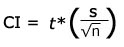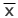# Calculating Sample Size for Task Times (Continuous Method)

Jeff Sauro, PhDWe already saw how a manageable sample of users can provide meaningful data for discrete-binary data like task completion. With continuous data like task times, the sample size can be even smaller.

The continuous calculation is a bit more complicated and involves somewhat of a Catch-22. Most want to determine the sample size ahead of time, then perform the testing based on the results of the sample size calculation as in the binary sample calculation. In this case, we need to have some data already or at least a strong hypothesis of our user population.

As with the binary calculation for task completion, we know when testing experienced users (those who complete the task at least weekly) they should overwhelmingly complete the task successfully. With task times we should also have a rough estimate of the mean and standard deviation ( there’s the Catch 22). If you’re performing a benchmarking study and already have some data, then you can use that data. If you have time, sample a pretest of users, say four, to get a sense of the range in times. Of course when all else fails you can have some internal folks complete the tasks–perhaps some sales or service employees or whomever comes close to matching the speed and accuracy of you target users. You’ll need to have an idea of the standard deviation(in seconds) for each task you’re testing.

For example, lets use the sample task, “Looking up a balance on an account number” (a very common task in accounting software). You write up a scenario and try the task yourself and have three of you colleagues complete it. Chances are you’re probably completing the task faster than your users, nevertheless it will still provide you a range of times. Here are the times in seconds

 Time (in seconds) You 101 Colleague 1 132 Colleague 2 125 Colleague 3 145 Mean 125.75 St Deviation 18.46 Range 44

From this pre-test sample you want to be able to derive as close an estimate as possible to the range in times of your actual users. To operationalize this, you would say “I want to be 95% confident of the mean time within ten seconds. So instead of simply asking, “How many users do I need to test?”, you ask “How many users do I need to test to be 95% sure I know their mean task time within ten seconds?” Here’s where the real statistics start.

That ten second range will become the confidence interval. The confidence interval is that + or – fudge factor seen with the polls on TV. With this confidence interval we can work backwards to arrive at our sample size. Because we don’t know the standard deviation of the whole population of users(again the Catch 22) we need to estimate it from the small sample we have. For small samples (less than 30) where the parent standard deviation (σ) is not known you use what’s called the student t distribution. The student t distribution uses values from a t table instead of the more familiar z table of normal values.

The confidence interval is calculated by multiplying this t-statistic (t*) by the Standard Error (SE). The Standard Error is just the sample standard deviation divided by the square root of the sample size. So the confidence interval formula usually looks something like this:To arrive at the elusive “significant” sample size, you need to try a few reasonable sample sizes and see which ones fall within the limits of the confidence interval. The values (n) you choose will affect the the critical value for t and the Standard Error since both use n in their equation. We’ll use 25, 20, 15, 10 and 5 and which ever value has a confidence interval at about 10 seconds we’ll use as the ideal sample. (Again all this assumes that our internal sample did a good job of determining the standard deviation of the larger population).

At about 15 users, the conifdence interval narrows close enough to ten seconds that it will probably be sufficient. I’d use this 15 as the approximate number of users you’d need to sample and know that to get more precise, you’d need to sample more than 15 users. This result is much better than thinking you need to test 100 or 1000 in order to get “statistically significant results. If +/- 10 seconds isn’t precise enough you can:

1. Decrease your confidence level to 90% or 85%.
2. Sample more users.

Sample Sizes in the Real World of Usability Testing

If you’ve run enough usability tests, in many cases your sample size is usually determined ahead of time–that is, you know your budget and time frame and therefore approximately how many users you’ll be sampling–usually somewhere between 10 and 30. I then approach sampling as getting as many users as I can within that range and then compute the statistics later.

For example, lets say we followed our initial indication and sampled 15 users (assuming our budget and time fit nicely with this figure). We had them complete the same task of looking up an account balance as our small internal employee sample. Here are the results next to our initial internal sample:

 Real-Users Sample User Time (in seconds) 1 101 2 140 3 112 4 144 5 132 6 99 7 118 8 125 9 154 10 115 11 118 12 125 13 141 14 145 15 130 Mean 126.6 St Deviation 16.33 Range 55
 Internal Sample Time You 101 Colleague 1 132 Colleague 2 125 Colleague 3 145 Mean 125.75 St Dev 18.46 Range 44

With this sample we can now estimate the true mean time of our population. Using the formula for the student t distribution:mean time of your sample (126.6)true mean time of the entire population of users n number of users in the sample (15) s the standard deviation of the sample (16.33) t* t statistic = (2.144789) or use the excel function =TINV(.05,14) [confidence level(.05) and degrees of freedom n-1 (14) ]

Plugging in the numbers, for the estimated mean of the total population of users on this task we get:= 126.6 + or – 9.08

So when reporting the mean time for this task we would say, “We are 95% confident the mean time is between 117.5 seconds and 135.6 seconds.” In this example, our original sample turned out to be a good estimate of the mean time and standard deviation but don’t expect that to usually work out so well.

0
0